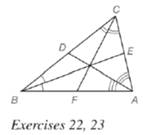Chapter 5.6, Problem 22EElementary Geometry For College St...

7th Edition
Alexander + 2 others
ISBN: 9781337614085

Solutions

Chapter
SectionElementary Geometry For College St...

7th Edition
Alexander + 2 others
ISBN: 9781337614085
Textbook Problem

In Δ A B C , m ∠ C A B = 80 ∘ , m ∠ A C B = 60 ∘ ,  and  ∠ A B C = 40 ∘ . With the angle bisectors as shown, which line segment is longer? a) A E ¯ or E C ¯ ? b) C D ¯ or D B ¯ ? c) A F ¯ or F B ¯ ?To determine

a)

To find:

The longer line segment, AE¯ or EC¯.

Explanation

Given:

In ΔABC, mCAB=80,mACB=60,mABC=40.

Theorem used:

Angle bisector theorem:

If a ray bisects one angle of a triangle, then it divides the opposite side into segments whose lengths are proportional to the lengths of the two sides that form the bisected angle.

Calculation:

Given that mCAB=80,mACB=60,mABC=40

To determine

b)

To find:

The longer line segment, CD¯ or DB¯.

To determine

c)

To find:

The longer line segment, AF¯ or FB¯.

Still sussing out bartleby?

Check out a sample textbook solution.

See a sample solution

The Solution to Your Study Problems

Bartleby provides explanations to thousands of textbook problems written by our experts, many with advanced degrees!

Get Started

Evaluate each expression: 6+2(542)

Elementary Technical Mathematics

23. (a) Graph . (b) Graph . (c) Algebraically show why these graphs are identical.

Mathematical Applications for the Management, Life, and Social Sciences

Describe the scores in a sample that has standard deviation of zero.

Statistics for The Behavioral Sciences (MindTap Course List)

Add the following numbers.

Contemporary Mathematics for Business & Consumers

What is the integrating factor for xy′ + 6x2y = 10 − x3?

Study Guide for Stewart's Single Variable Calculus: Early Transcendentals, 8th